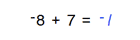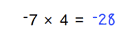# Home | Mathematics | Pre-Algebra

## Solving Binomials

Binomial equations are the easiest way to start solving equations because they involve only one basic math operation. They are only a little different from basic math problems where a parameter is missing instead of the operation's result. This worksheet will help students become accustomed to solving problems containing variables.

## Solving Binomials Options:

 Values size: up to 20 or 40   up to 40 or 80   up to 50 or 130   up to 100 or 600   up to 200 or 2500 Operations:AdditionSubtractionMultiplicationDivision Paper size: US Letter (8.5 x 11 in)   A4 (210 x 297 mm)